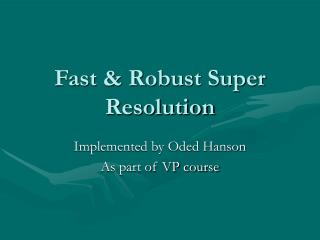DownloadDownload PresentationFast & Robust Super Resolution

# Fast & Robust Super Resolution

Download Presentation## Fast & Robust Super Resolution

- - - - - - - - - - - - - - - - - - - - - - - - - - - E N D - - - - - - - - - - - - - - - - - - - - - - - - - - -
##### Presentation Transcript

1. Fast & Robust Super Resolution Implemented by Oded Hanson As part of VP course

2. What is super-resolution • A means of producing a high-resolution image from a set of low-resolution, blurred and noisy images. • The means in achieving this HR image is usually done by estimating an image which minimizes some cost of difference of its projection to each of the LR images.

3. Least-Squares Estimation • When the noise added to the LR images is white (normally distributed with zero mean) then it is known that the least squares approach is the ML (Maximum Likelihood) estimator and is efficient (Optimal in the sense of MVU). • Since the number of variables to estimate is extremely large (the number of pixels in the HR image), the cost function is usually regulated with some prior-knowledge transforming this process to a MAP (Maximum A-Post priory) Estimation.

4. Robust Estimation • What happens when the noise isn’t exactly white? • Actually the breakdown factor of the least-squares approach is 1. That is, a single outlier can ruin the entire estimation process. • L1 Norm estimation is not efficient, that is not a MVU, but has an breakdown factor of 50% and often out-performs the so-called “optimal” least squared.

5. Fast and Robust Multi-frame Super Resolution • This is a work performed by Sina Farsiu together with M. Elad, P. Milanfar and D. Robinson. • In this work they suggested two methods for robust super-resolution by minimizing an L1 energy function, and using the bilateral filter as a regulating term. • The first method is general and will work for any type of motion. • The second method is extremely fast, but is only appropriate for translational motion.

6. What did I do in this work • In this work I implemented the two methods suggested in the paper. • I implemented an optical-flow based registration function • I implemented a Graphical User Interface for evaluating the performance of the implementations.